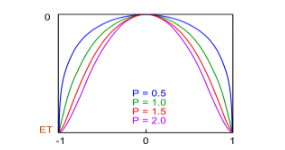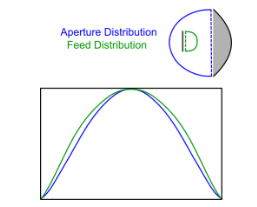#### Aperture distribution calculatorThis calculator plots the approximate aperture and feed distribution of a typical axissymetrical parabolic reflector topology. The feed / aperture distribution is determined based on the the shape modifier (P) derived for the given focal length to diameter ratio (F/D), edge taper (ET) and aperture / feed distribution efficiency (ade / fde) [Rahmat-Samii].

The above image shows the effect of shape modifier P on the feed / aperture distribution.

The distribution at the feed is different to the distribution at the aperture, due to the properties of the main reflector. Depending on the F/D ratio of the dish, the graph below may vary. The aperture or feed efficiency is calculated relative to an 'ideal' flat distribution.Image of aperture vs feed distribution